<![CDATA[Billy Ian's Short Leisure-time Wander]]> 2021-10-17T13:39:10-04:00 http://billy-inn.github.io/ Octopress <![CDATA[Notes on Convex Optimization (5): Newton's Method]]> 2018-11-13T23:25:12-05:00 http://billy-inn.github.io/blog/2018/11/13/convex-optimization-5 For $x\in\mathbf{dom}\ f$, the vector

is called the Newton step (for $f$, at $x$).

#### Minimizer of second-order approximation

The second-order Taylor approximation $\hat f$ of $f$ at $x$ is

\begin{equation} \hat f(x+v) = f(x) + \nabla f(x)^T v + \frac12 v^T \nabla^2 f(x) v. \tag{1} \label{eq:1} \end{equation}

which is a convex quadratic function of $v$, and is minimized when $v=\Delta x_{nt}$. Thus, the Newton step $\Delta x_{nt}$ is what should be added to the point $x$ to minimize the second-order approximation of $f$ at $x$.

#### Steepest descent direction in Hessian norm

The Newton step is also the steepest descent direction at $x$, for the quadratic norm defined by the Hessian $\nabla^2 f(x)$, i.e.,

#### Solution of linearized optimality condition

If we linearize the optimality condition $\nabla f(x^*)=0$ near $x$ we obtain

which is a linear equation in $v$, with solution $v=\Delta x_{nt}$. So the Newton step $\Delta x_{nt}$ is what must be added to $x$ so that the linearized optimality condition holds.

#### Affine invariance of the Newton step

An important feature of the Newton step is that it is independent of linear changes of coordinates. Suppose $T\in \mathbf{R}^{n \times n}$ is nonsingular, and define $\bar f(y)=f(Ty)$. Then we have

where $x=Ty$, likewise we have $\nabla^2 \bar f(y) = T^T\nabla^2f(x)T$. The Newton step for $\bar f$ at $y$ is therefore

where $\Delta x_{nt}$ is the Newton step for $f$ at $x$. Hence the Newton steps of $f$ and $\bar f$ are related by the same linear transformation, and

#### The Newton decrement

The quantity

is called the Newton decrement at $x$. We can relate the Newton decrement to the quantity $f(x) - \inf_y \hat f(y)$, where $\hat y$ is the second-order approximation of $f$ at $x$:

We can also express the Newton decrement as

This shows that $\lambda$ is the norm of the Newton step, in the quadratic norm defined by the Hessian.

### Newton’s Method

Newton’s method.
given a starting point $x \in \mathbf{dom} \enspace f$, tolerance $\epsilon > 0$.
repeat
- Compute the Newton step $\Delta x_{nt}$ and decrement $\lambda^2$.
- Stopping criterion. quit** if $\lambda(x)^2/2 \le \epsilon$.
- *Line search
. Choose a step size $t > 0$ by backtracking line search.
- Update. $x := x+ t\Delta x_{nt}$.

### Summary

Newton’s method has several very strong advantages over gradient and steepest descent methods:

• Convergence of Newton’s method is rapid in general, and quadratic near $x^\ast$. Once the quadratic convergence phase is reached, at most six or so iterations are required to produce a solution of very high accuracy.
• Newton’s method is affine invariant. It is insensitive to the choice of coordinates, or the condition number of the sublevel sets of the objective.
• The good performance of Newton’s method is not dependent on the choice of algorithm parameters. In contrast, the choice of norm for steepest descent plays a critical role in its performance.

The main disadvantage of Newton’s method is the cost of forming and storing the Hessian, and the cost of computing the Newton step, which requires solving a set of linear equations.

]]>
<![CDATA[Notes on Convex Optimization (4): Gradient Descent Method]]> 2018-11-05T00:29:05-05:00 http://billy-inn.github.io/blog/2018/11/05/convex-optimization-4 Descent methods
• $f(x^{(k+1)}) < f(x^{(k)})$
• $\Delta x$ is the step or search direction; $t$ is the step size or step length
• from convexity, $\nabla f(x)^T \Delta x <0$

General descent method.
given a starting point $x \in \mathbf{dom} \enspace f$.
repeat
- Determine a descent direction $\Delta x$.
- Line search. Choose a step size $t > 0$.
- Update. $x := x+ t\Delta x$.

until stopping criterion is satisfied

Backtracking line search.
given a descent direction $\Delta x$ for $f$ at $x \in \mathbf{dom} f, \alpha \in(0,0.5), \beta\in(0,1)$.
starting at $t:=1$.
while $f(x+t\Delta x) > f(x) + \alpha t \nabla f(x)^T \Delta x$, $t:=\beta t$

A natural choice for the search direction is the negative gradient $\Delta x = - \nabla f(x)$.

We must have $f(x^(k)) - p^\ast \le \epsilon$ after at most

iterations of the gradient method with exact line search, where $c=1-m/M<1$.

Similar to exact line search, except that $c=1 - \min{2m\alpha, 2\beta\alpha m/M} < 1.$

#### Conclusions

• The gradient method often exhibits approximately linear convergence, i.e., the error $f(x^{(k)}) - p^\ast$ converges to zeros approximately as a geometric series.
• The choice of backtracking parameters $\alpha, \beta$ has a noticeable but not dramatic effect on the convergence. An exact line search sometimes improves the convergence of the gradient method, but the effect is not large.
• The convergence rate depends greatly on the condition number of the Hessian, or the sublevel sets. Convergence can be very slow, even for problems that are moderately well conditioned. When the condition number is larger the gradient method is so slow that it is useless in practice.

### Steepest descent method

The first-order Taylor approximation of $f(x+v)$ around $x$ is

The second term on the righthand side, $\nabla f(x)^T v$, is the directional derivative of $f$ at $x$ in the direction $v$. It gives the approximate change in $f$ for a small step $v$. The step $v$ is a descent direction if the directional derivative is negative.

Let $\lVert \cdot \rVert$ be any norm on $\mathbf{R}^n$. We define a normailzied steepest descent direction as

\begin{equation} \Delta x_{nsd} = \arg\min{\nabla f(x)^T v\ \vert\ \lVert v \rVert = 1}. \tag{1}\label{eq:1} \end{equation}

It is also convenient to consider a steepest descent step $\Delta x_{sd}$ that is unnormalized, by scaling the normalized steepest descent direction in a particular way:

\begin{equation} \Delta x_{sd} = \lVert \nabla f(x) \rVert_\ast \Delta x_{nsd}, \tag{2}\label{eq:2} \end{equation}

where $\lVert \cdot \rVert_\ast$ denotes the dual norm. Note that for the steepest descent step, we have

#### Steepest descent for Euclidean norm

To simplify the notation, we can look at the problem of solving $\min_v{u^Tv\ \lvert\ \lVert v \rVert \le 1}$ which ends up being equivalent to find the normalized steepest descent step.

The Cauchy-Schwarz inequality gives $\lvert u^Tv\rvert \le \rVert u \rVert \lVert v \rVert$, hence it is easy to see that the minimum is $\min_v{u^Tv\ \lvert\ \lVert v \rVert \le 1}=-\lVert u \rVert$, and the minimizer is $v=-u/\lVert u \rVert$. As a result, the steepest descent direction is simply the negative gradient, i.e., $\Delta x_{sd} = - \nabla f(x)$.

#### Steepest descent for quadratic norm

where $P \in \mathbf{S}_{++}^n$. The problem is now $\min_v{u^Tv\ \vert\ \lVert P^{1/2}v\rVert\le1}=\min_v{u^Tv\ \vert\ \lVert\delta\rVert\le1, v=P^{-1/2}\delta}$. This is equivalent to $\min_\delta{((P^{-1/2})^Tu)^T\delta\ \vert\ \lVert\delta\rVert\le1}$. The problem above shows that the minimum is $-\lVert (P^{-1/2})^Tu\rVert$ while the maximum $\lVert (P^{-1/2})^Tu\rVert$ is the dual norm according to the definition, and the minimizer is $v=P^{-1/2}\delta=-P^{-1}u/\lVert (P^{-1/2})^Tu\rVert$, so the steepest descent desnt is given by

In addition, the steepest descent method in the quadratic norm $\lVert \cdot \rVert_P$ can be thought of as the gradient method applied to the problem after the change of coordinates $\bar x=P^{1/2}x$.

#### Steepest descent for $l_1$-norm

Let $i$ be any index for which $\lVert \nabla f(x) \rVert_\infty = \lvert (\nabla f(x))_i \rvert$. Then a normalized steepest descent direction $\nabla x_{nsd}$ for the $l_1$-norm is given by

where $e_i$ is the $i$th standard basis vector. An unnormalized steepest descent step is then

The steepest descent algorithm in the $l_1$-norm has a very natural interpertation: At each iteration we select a component of $\nabla f(x)$ with maximum absolute value, and then decrease or increase the corresponding component of $x$, according to the sign of $(\nabla f(x))_i$. The algorithm is sometimes called a corrdinate-descent algorithm, since only one component of the variable $x$ is updated at each iteration.

]]>
<![CDATA[Notes on Convex Optimization (3): Unconstrained Minimization Problems]]> 2018-09-29T15:15:12-04:00 http://billy-inn.github.io/blog/2018/09/29/notes-on-convex-optimization-3-unconstrained-minimization-problems Unconstrained optimization problems are defined as follows:

\begin{equation} \text{minimize}\quad f(x) \tag{1} \label{eq:1} \end{equation}

where $f: \mathbf{R}^n \rightarrow \mathbf{R}$ is convex and twice continously differentiable (which implies that $\mathbf{dom}\enspace f$ is open). We denote the optimal value $\inf_xf(x)=f(x^\ast)$, as $p^\ast$. Since $f$ is differentiable and convex, a necessary and sufficient condition for a point $x^\ast$ to be optimal is

\begin{equation} \nabla f(x^\ast)=0. \tag{2} \label{eq:2} \end{equation}

Thus, solving the unconstrained minimization problem \eqref{eq:1} is the same as finding a solution of \eqref{eq:2}, which is a set of $n$ equations in the $n$ variables $x_1, \dots, x_n$. Usually, the problem must be solved by an iterative algorithm. By this we mean an algorithm that computes a sequence of points $x^{(0)}, x^{(1)}, \dots \in \mathbf{dom}\enspace f$ with $f(x^{(k)})\rightarrow p^\ast$ as $k\rightarrow\infty$. The algorithm is terminated when $f(x^{k}) - p^\ast \le \epsilon$, where $\epsilon>0$ is some specified tolerance.

#### Initial point and sublevel set

The starting point $x^{(0)}$ must lie in $\mathbf{dom}\enspace f$, and in addition the sublevel set

must be closed. This condition is satisfied for all $x^{(0)}\in\mathbf{dom}\enspace f$ if the function $f$ is closed.

Note: 1) Continuous functions with $\mathbf{dom}\enspace f=\mathbf{R}^n$ are closed; 2) Another important class of closed functions are continuous functions with open domains.

### Examples

The general convex quadratic minimization problem has the form

where $P\in\mathbf{S}_+^n$, $q\in\mathbf{R}^n$, and $r\in\mathbf{R}$. This problem can be solved via the optimality conditions, $Px+q=0$, which is a set of linear equations.

One special case of the quadratic minimization problem that arises very frequently is the least-squares problem

The optimality condition

are called the normal equations of the least-squares problem.

#### Analytic center of linear inequalities

where the domain of $f$ is the open set

#### Analytic center of a linear matrix inequality

where $F: \mathbf{R}^n\rightarrow\mathbf{S}^p$ is affine. Here the domain of $f$ is

### Strong convexity and implications

The objective function is strongly convex on $S$, which means that there exists an $m>0$ such that

\begin{equation} \nabla^2f(x) \succeq mI \tag{3} \label{eq:3} \end{equation}

for all $x\in S$. For $x, y \in S$ we have

for some $z$ on the line segement $[x, y]$. By the strong convexity assumption \eqref{eq:3}, the last term on the righthand side is at least $(m/2)\lVert y-x\rVert^2_2$, so we have the inequality

\begin{equation} f(y) \ge f(x) + \nabla f(x)^T(y-x) + \frac{m}2\lVert y-x \rVert_2^2 \tag{4} \label{eq:4} \end{equation}

for all $x$ and $y$ in $S$.

#### Bound $f(x)-p^\ast$ in terms of $\lVert \nabla f(x) \rVert_2$

Setting the gradient of the righthand side of \eqref{eq:4} with respect to $y$ equal to zero, we find that $\tilde y = x-(1/m)\nabla f(x)$ minimizes the righthand side. Therefore we have

Since this holds for any $y\in S$, we have

\begin{equation} p^* \ge f(x) - \frac1{2m}\lVert \nabla f(x)\rVert_2^2 \tag{5} \label{eq:5} \end{equation}

This inequality shows that if the gradient is small at a point, then the point is nearly optimal.

#### Bound $\lVert x-x^\ast\rVert_2^2$ in terms of $\lVert \nabla f(x) \rVert_2$

Apply \eqref{eq:4} with $y=x^\ast$ to obtain

where we use the Cauchy-Schwarz inequality in the second inequality. Since $p^\ast \le f(x)$, we must have

Therefore, we have

\begin{equation} \lVert x - x^\ast \rVert_2 \le \frac2m\lVert \nabla f(x) \rVert_2. \tag{6}\label{eq:6} \end{equation}

#### Uniqueness of the optimal point $x^\ast$

If there are two optimal point $x^\ast_1, x^\ast_2$, according to \eqref{eq:6},

Hence, $x_1^\ast = x_2^\ast$, the optimal point $x^\ast$ is unique.

#### Upper bound on $\nabla^2f(x)$

There exists a constant $M$ such that

for all $x \in S$. This upper bound on the Hessian implies for any $x, y \in S$,

minimizing each side over $y$ yields

#### Condition number of sublevel sets

The ratio $\kappa=M/m$ is an upper bound on the condition number of the matrix $\nabla^2 f(x)$, i.e., the ratio of its largest eigenvalue to its smallest eigenvalue.

We define the width of a convex set $C \subseteq \mathbf{R}^n$, in the direction $q$, where $\lVert q \rVert_2 = 1$, as

The minimum width and maximum width of $C$ are given by

The condition number of the convex set $C$ is defined as

Suppose $f$ satisfies $mI \preceq \nabla^2 f(x) \preceq MI$ for all $x\in S$. The condition number of the $\alpha$-sublevel $C_\alpha={x \vert f(x) \le \alpha}$, where $p^\ast < \alpha \le f(x^{(0)})$, is bounded by

#### The strong convexity constants

It must be kept in mind that the constants $m$ and $M$ are known only in rare cases, so they cannot be used in a practical stopping criterion.

]]>
<![CDATA[[Notes on Mathematics for ESL] Chapter 10: Boosting and Additive Trees]]> 2017-12-14T19:07:57-05:00 http://billy-inn.github.io/blog/2017/12/14/esl-chapter10 10.5 Why Exponential Loss?

#### Derivation of Equation (10.16)

Since $Y\in{-1,1}$, we can expand the expectation as follows:

In order to minimize the expectation, we equal derivatives w.r.t. $f(x)$ as zero:

which gives:

#### Notes on Equation (10.18)

If $Y=1$, then $Y’=1$, which gives

Likewise, if $Y=-1$, then $Y’=0$, which gives

As a result, the binomial log-likelihood loss is equivalent to the deviance. In the language of neural networks, the cross-entropy is equivalent to the softplus. The only difference is that $0$ is used to indicate negative examples in cross-entropy; while $-1$ is used in softplus.

### 10.6 Loss Functions and Robustness

This section explains the choice of loss functions for both classification and regression. It gives a very direct expalanation about why square loss is undesirable for classification. Highly recommended!

]]>
<![CDATA[[Notes on Mathematics for ESL] Chapter 6: Kernel Smoothing Methods]]> 2017-10-27T18:57:21-04:00 http://billy-inn.github.io/blog/2017/10/27/esl-chapter-6 6.1 One-Dimensional Kernel Smoothers

#### Notes on Local Linear Regression

Locally weighted regression solves a separate weighted least squares problem at each target point $x_0$:

The estimate is $\hat f(x_0)=\hat\alpha(x_0)+\hat\beta(x_0)x_0$. Define the vector-value function $b(x)^T=(1,x)$. Let $\mathbf{B}$ be the $N \times 2$ regression matrix with $i$th row row $b(x_i)^T$, $\mathbf{W}(x_0)$ the $N\times N$ diagonal matrix with $i$th diagonal element $K_\lambda (x_0, x_i)$, and $\theta=(\alpha(x_0), \beta(x_0))^T$.

Then the above optimization problem can be rewritten as

Equal the derivative w.r.t $\theta$ as zero, we get

Then

It’s claimed that $\sum_{i=1}^Nl_i(x_0)=1$ and $\sum_{i=1}^N(x-x_0)l_i(x_0)=0$ in the book, so that the bias $\text{E}(\hat f(x_0))-f(x_0)$ depends only on quadratic and higher-order terms in the expansion of $f$. However, the proof is not given. Here I will give the detailed derivations of these two equations.

First, define the following terms:

Then, we can represent the estimate as

When $y=\mathbf{1}$, $m_0=S_0$ and $m_1=S_1$, we get

When $y=\mathbf{x}-x_0$,

More generally, it’s easy to show that $\sum_{i=1}^N(x_i-x_0)^pl_i(x_0)=0$ when $p>0$.

We only prove the case when the input $x$ is one-dimensional. Similar strategy can be used to prove the case for high-dimensional input, but it’ll be a little bit complicated if you’re interested. Have fun!

]]>
<![CDATA[[Notes on Mathematics for ESL] Chapter 5: Basis Expansions and Regularization]]> 2017-10-24T20:34:25-04:00 http://billy-inn.github.io/blog/2017/10/24/esl-chapter-5 5.4 Smoothing Splines

#### Derivation of Equation (5.12)

Equal the derivative of Equation (5.11) as zero, we get

Put the terms related to $\theta$ on one side and the others on the other side, we get

Multiply the inverse of $N^TN+\lambda\Omega_N$ on both sides completes the derivation of Equation (5.12)

#### Explanations on Equation (5.17) and (5.18)

It’s a little confusing to get Equation (5.18) directly from Equation (5.17) and its original form Equation (5.11). In order to give a clear explanation, here we give the proof of the equation,

which are the different terms between Equation (5.11) and Equation (5.18).

We know that

following Equation (5.14) in the book.

From Equation (5.17), we can get

Plug the above two equation into the right side of the equation remains to be proved, we get

which completes the proof.

]]>
<![CDATA[Notes on Convex Optimization (2): Convex Functions]]> 2017-10-21T13:59:09-04:00 http://billy-inn.github.io/blog/2017/10/21/convex-optimization-2 1. Basic Properties and Examples

#### 1.1 Definition

$f:\mathbb{R}^n \rightarrow \mathbb R$ is convex if $\mathbf{dom}\ f$ is a convex set and

for all $x,y\in \mathbf{dom}\ f, 0\le\theta\le1$

• $f$ is concave if $-f$ is convex
• $f$ is strictly convex if $\mathbf{dom}\ f$ is convex and $% <![CDATA[ f(\theta x+(1-\theta)y)<\theta f(x)+(1-\theta)f(y) %]]>$ for $x,y\in\mathbf{dom}\ f,x\ne y, 0<\theta<1$

$f:\mathbb{R}^n \rightarrow \mathbb R$ is convex if and only if the function $g: \mathbb{R} \rightarrow \mathbb{R}$,

is convex (in $t$) for any $x \in \mathbf{dom}\ f, v\in\mathbb R^n$

#### 1.2 Extended-value extensions

extended-value extension $\tilde f$ of $f$ is

#### 1.3 First-order conditions

1st-order condition: differentiable $f$ with convex domain is convex iff

#### 1.4 Second-order conditions

2nd-order conditions: for twice differentiable $f$ with convex domain - $f$ if convex if and only if $\nabla^2f(x)\succeq0\mathrm{\ for\ all\ } x\in\mathbf{dom}\ f$ - if $\nabla^2f(x)\succ0$ for all $x\in\mathbf{dom}\ f$, then $f$ is strictly convex

#### 1.5 Sublevel sets and epigraph

$\alpha$-sublevel set of $f: \mathbb R^n \rightarrow \mathbb R$:

sublevel sets of convex functions are convex (converse if false)

If $f$ is concave, then its $\alpha$-superlevel set, given by ${x\in\mathbf{dom}\ f\mid f(x)\le\alpha}$, is a convex set

epigraph of $f:\mathbb R^n \rightarrow \mathbb R$:

$f$ is convex if and only if $\mathbf{epi}\ f$ is a convex set

$f$ is concave if and only if its hypograph, defined as

is a convex set

#### 1.6 Jensen’s inequality and extensions

Jensen’s Inequality: if $f$ is convex, then for $0\le\theta\le1$,

extension: if $f$ is convex, then

for any random variable $z$

### 2. Operations that Preserve Convexity

#### 2.1 Positive weighted sum & composition with affine function

nonnegative multiple: $\alpha f$ is convex if $f$ is convex, $\alpha \ge 0$

sum: $f_1+f_2$ convex if $f_1,f_2$ convex (extends to infinite sums, integrals)

composition with affine function: $f(Ax+b)$ is convex if $f$ is convex

#### 2.2 Pointwise maximum

pointwise maximum: if $f_1,\dots,f_m$ are convex, then $f(x)=\max{f_1(x),\dots,f_m(x)}$ is convex

pointwise supermum: if $f(x,y)$ is convex in $x$ for each $y\in\mathcal{A}$, then

is convex

similarly, the pointwise infimum of a set of concave functions is a concave function

#### 2.3 Composition

composition with scalar functions: composition of $g: \mathbb R^n \rightarrow \mathbb R$ and $h: \mathbb R\rightarrow \mathbb R$:

$f$ is convex if $g$ convex, $h$ convex, $\tilde h$ nondecreasing; $g$ concave, $h$ convex, $\tilde h$ nonincreasing

Note: monotonicity must hold for extended-value extension $\tilde h$

vector composition: composition of $g:\mathbb R^n \rightarrow \mathbb R^k$ and $h:\mathbb R^k \rightarrow \mathbb R$:

$f$ is convex if $g_i$ convex, $h$ convex, $\tilde h$ nondecreasing in each argument; $g$ concave, $h$ convex, $\tilde h$ nonincreasing in each argument

#### 2.4 Minimization

minimization: if $f(x,y)$ is convex in $(x,y)$ and $C$ is a convex set then

is convex

#### 2.5 Perspective of a function

perspective: the perspective of a function $f:\mathbb R^n \rightarrow \mathbb R$ is the function $g:\mathbb R^n \times \mathbb R \rightarrow \mathbb R$,

$g$ is convex if $f$ is convex

### 3. The Conjugate Function

#### 3.1 Definition

the conjugate of a function $f$ is

• $f^*$ is convex whether or not $f$ is convex

#### 3.2 Basic properties

conjugate of the conjugate: if $f$ is convex and closed, then $f^{**}=f$

differentiable functions: The conjugate of a differentiable function $f$ is also called the Legendre transform of $f$. Let $z\in\mathbb{R}^n$ be arbitrary and define $y=\nabla f(z)$, then we have

scaling and composition with affline transformation: For $a>0$ and $b\in\mathbb{R}$, the conjugate of $g(x)=af(x)+b$ is $g^*(y)=af^*(y/a)-b$.

Suppose $A\in\mathbb{R}^{n\times n}$ is nonsingular and $b\in\mathbb{R}^n$. Then the conjugate of $g(x)=f(Ax+b)$ is

with $\mathbf{dom}\ g^*=A^T\mathbf{dom}\ f^*$

sums of independent functions: if $f(u,v)=f_1(u)+f_2(v)$, where $f_1$ and $f_2$ are convex functions with conjugates $f_1^*$ and $f_2^*$, respectively, then

### 4. Quasiconvex Functions

$f:\mathbb R^n\rightarrow \mathbb R$ is quasiconvex if $\mathbf{dom}\ f$ is convex and the sublevel sets

are convex for all $\alpha$

• $f$ is quasiconcave if $-f$ is quasiconvex
• $f$ is quasilinear if it is quasiconvex and quasiconcave
• convex functions are quasiconvex, but the converse is not true

modified Jensen inequality: for quasiconvex $f$

first-order condition: differentiable $f$ with convex domain is quasiconvex iff

operations that preserve quasiconvexity:

• nonnegative weighted maximum
• composition
• minimization

sum of quasiconvex functions are not necessarily quasiconvex

### 5. Log-concave and Log-convex Functions

a positive function $f$ is log-concave if $\log f$ is concave:

$f$ is log-convex if $\log f$ is convex

properties of log-concave functions:

• twice differentiable $f$ with convex domain is log-concave iff

for all $x\in\mathbf{dom}\ f$

• product of log-concave functions is log-concave
• sum of log-concave functions is not always log-concave; however, log-convexity is preserved under sums
• integration: if $f:\mathbb R^n\times\mathbb R^m \rightarrow \mathbb R$ is log-concave, then

is log-concave

consequences of integration property:

• convolution $f*g$ of log-concave functions $f,g$ is log-concave
• if $C\subseteq \mathbb R^n$ convex and $y$ is a random variable with log-concave pdf then

is log-concave

### 6. Convexity w.r.t. Generalized Inequalities

$f:\mathbb{R}^n\rightarrow\mathbb{R}^m$ is $K$-convex if $\mathbf{dom}\ f$ is convex and

for $x,y\in\mathbf{dom}\ f,0\le\theta\le1$

]]>
<![CDATA[Notes on Convex Optimization (1): Convex Sets]]> 2017-10-17T02:22:23-04:00 http://billy-inn.github.io/blog/2017/10/17/convex-optimization-1 1. Affine and Convex Sets

Suppose $x_1\ne x_2$ are two points in $\mathbb{R}^n$.

#### 1.1 Affine sets

line through $x_1$, $x_2$: all points

affine set: contains the line through any two distinct points in the set

#### 1.2 Convex sets

line segment between $x_1$ and $x_2$: all points

with $0\leq\theta\leq1$

convex set: contains line segment between any two points in the set

convex combination of $x_1,\dots,x_k$: any point $x$ of the form

with $\theta_1+\dots+\theta_k=1,\theta_i \geq 0$

convex hull of a set $C$, denoted $\mathbf{conv}\ C$: set of all convex combinations of points in $C$

#### 1.3 Cones

conic combination of $x_1$ and $x_2$: any point of the form

with $\theta_1 \geq 0, \theta_2 \geq 0$

convex cone: set that contains all conic combinations of points in the set

### 2. Some Important Examples

#### 2.1 Hyperplanes and halfspaces

hyperplane: set of the form {$x\mid a^Tx=b$}$(a\ne0)$

halfspace: set of the form {$x\mid a^Tx\leq b$}$(a\ne0)$

• $a$ is the normal vector
• hyperplanes are affine and convex; halfspaces are convex

#### 2.2 Euclidean balls and ellipsoids

(Euclidean) ball with center $x_c$ and radius $r$:

ellipsoid: set of the form

with $P\in \mathbf{S}^n_{++}$ (i.e., P symmetric positive definite)

another representation: {$x_c+Au\mid \lVert u\rVert_2\le1$} with $A$ square and nonsingular

• Euclidean balls and ellipsoids are all convex.

#### 2.3 Norm balls and norm cones

norm: a funtion $\lVert \centerdot \rVert$ that satisfies

• $\lVert x \rVert \geq 0$; $\lVert x \rVert=0$ if and only if $x=0$
• $\lVert tx \rVert = \lvert t \rvert \lVert x \rVert$ for $t\in \mathbb{R}$
• $\lVert x+y\rVert \leq \lVert x \rVert+\lVert y \rVert$

norm ball with center $x_c$ and radius $r: \{x \mid \lVert x-x_c \rVert \leq r\}$

norm cone: $\{(x,t) \mid \lVert x \rVert \leq t\}$

• norm balls and cones are convex
• norm cores (as the name suggest) are convex cones

#### 2.4 Polyhedra

polyhedra: solution set of finitely many linear inequalities and equalities

($A\in \mathbb{R}^{m\times n}$, $C\in\mathbb{R}^{p\times n}$, $\preceq$ is componentwise inequality)

• polyhedron is intersection of finite number of halfspaces and hyperplances

#### 2.5 The positive semidefinite cone

positive semidefinite cone:

• $\mathbf{S}^n$ is set of symmetric $n\times n$ matrices
• $\mathbf{S}^n_+=\{X\in\mathbf{S}^n\mid X\succeq0\}$: positive semidefinite $n\times n$ matrices $X\in\mathbf{S}^n_+ \iff z^TXz \geq 0\ \mathrm{for\ all\ }z$ $\mathbf{S}^n_+$ is a convex cone
• $\mathbf{S}^n_{++}=\{X\in\mathbf{S}^n\mid X\succ0\}$: positive definite $n\times n$ matrices

### 3. Operations that preserve convexity

intersection: the interction of (any number of) convex sets is convex

affine function: suppose $f: \mathbb{R}^n \rightarrow \mathbb{R}^m$ is affine ($f(x)=Ax+b$ with $A\in\mathbb{R}^{m\times n}, b\in\mathbb{R}^m$)

• the image of a convex set under $f$ is convex
• the inverse image $f^{-1}(C)$ of a convex set under $f$ is convex

perspective function $P: \mathbb{R}^{n+1} \rightarrow \mathbb{R}^n$:

images and inverse images of convex sets under perspective are convex

linear-fractional function $f:\mathbb{R}^n \rightarrow \mathbb{R}^m$:

images and inverse images of convex sets under linear-fractional functions are convex

### 4. Generalized Inequalities

#### 4.1 Proper cones and generalized inequalities

a convex cone $K\subseteq\mathbb{R}^n$ is a proper cone if

• $K$ is closed (contains its boundary)
• $K$ is solid (has nonempty interior)
• $K$ is pointed (contains no line)

generalized inequality defined by a proper cone $K$:

#### 4.2 Minimum and minimal elements

$x\in S$ is the minimum element of $S$ with respect to $\preceq_K$ if

$x\in S$ is a minimal element of $S$ with respect to $\preceq_K$ if

### 5. Separating and Supporting Hyperplanes

separating hyperplane theorem: if $C$ and $D$ are disjoint convex sets, then there exists $a\ne0$, $b$ such that

supporting hyperplane to set $C$ at boundary point $x_0$:

where $a\ne0$ and $a^Tx\le a^Tx_0$ for all $x\in C$

supporting hyperplance theorem: if $C$ is convex, then there exists a supporting hyperplane at every boundary point of $C$

### 6. Dual Cones and Generalized Inequalities

#### 6.1 Dual cones

dual cone of a cone $K$:

Dual cons satisfy several properties, such as:

• $K^*$ is closed and convex
• $K_1 \subseteq K_2$ imples $K_2^* \subseteq K_1^*$
• $K^{**}$ is the closure of the convex hull of $K$ (Hence if $K$ is convex and closed, $K^{**}=K$)

Thsese properties show that if $K$ is a proper cone, then so is its dual $K^{*}$, and moreover, that $K^{**}=K$

#### 6.2 Dual generalized inequalities

dual cones of proper cones are proper, hence define generalized inequalities:

Some import properties relating a generalized inequality and its dual are:

• $x\preceq_K y$ iff $\lambda^Tx \le \lambda^Ty$ for all $\lambda \succeq_{K^{*}} 0$
• $x\prec_K y$ iff $\lambda^Tx < \lambda^Ty$ for all $\lambda \succ_{K^{*}} 0, \lambda\ne0$

Since $K=K^{**}$, the dual generalized inequality associated with $\preceq_{K^{*}}$ is $\preceq_K$, so these properties hold if the generalized inequality and its dual are swapped

#### 6.3 Minimum and minimal elements via dual inequalities

dual characterization of minimum element w.r.t. $\preceq_K$: $X$ is minimum element of $S$ iff for all $\lambda \succ_{K^*}0$, $x$ is the unique minimizer of $\lambda^Tz$ over $z\in S$

dual characterization of minimal element w.r.t. $\preceq_K$:

• if $x$ minimizes $\lambda^Tz$ over $S$ for some $\lambda \succ_{K^*}0$, then $x$ is minimal
• if $x$ is a minimal element of a convex set $S$, then there exists a nonzero $\lambda \succeq_{K^*}0$ such that $x$ minimizes $\lambda^Tz$ over $z \in S$
]]>
<![CDATA[[Notes on Mathematics for ESL] Chapter 4: Linear Methods for Classification]]> 2017-10-15T16:30:27-04:00 http://billy-inn.github.io/blog/2017/10/15/esl-chapter-4 4.3 Linear Discriminant Analysis

#### Derivation of Equation (4.9)

For that each class’s density follows multivariate Gaussian

Take the logarithm of $f_k(x)$, we get

where $c = -\log [(2\pi)^{p/2}\lvert\Sigma\rvert^{1/2}]$ and $\mu_k^T\Sigma^{-1}x=x^T\Sigma^{-1}\mu_k$. Following the above formula, we can derive Equation (4.9) easily

#### Notes on Computations for LDA

It’s stated in the book that the LDA classifier can be implemented by the following pair of steps:

• Sphere the data with respect to the common covariance estimate $\hat \Sigma:X^*\leftarrow D^{-1/2}U^TX$, where $\hat \Sigma=UDU^T$. The common covariance estimate of $X^*$ will now be the indentity.
• Classify to the closest class centroid in the transformed space, modulo the effect of the class prior probabilities $\pi_k$.

However, detailed explanation is not given in the book. Here, I give some skipped mathematical steps which may help the understanding.

which shows that the covariance estimate of $X^*$ is the identity.

Note that the classification for LDA is based on the linear discriminat functions

which is the Equation (4.10) in the book. Since the input $x$ is same for each class, so we can add back a term $\frac12x^T\Sigma^{-1}x$ which is cancelled in the previous derivation. Now the functions are turned into:

We know that $\Sigma=I$ in the transformed space, so $\delta_k(x)=-1/2\lVert x-\mu_k\rVert_2+\log\pi_k$. And $\mu_k$ is the centroid for the $k$th class. The claimed method to classify is proved.

### 4.4 Logistic Regression

#### Derivation of Equation (4.21) and (4.22)

In the two-class case, $p_1(x;\beta)=p(x;\beta)$ and $p_2(x;\beta) = 1-p(x;\beta)$ where

The Equation (4.21) can be derived easily as follows,

Note that

Plug it into Equation (4.21), we get

]]>
<![CDATA[[Notes on Mathematics for ESL] Chapter 3: Linear Regression Models and Least Squares]]> 2017-09-27T21:30:12-04:00 http://billy-inn.github.io/blog/2017/09/27/esl-chapter-3 3.2 Linear Regression Models and Least Squares

#### Derivation of Equation (3.8)

The least squares estimate of $\beta$ is given by the book’s Equation (3.6)

From the previous post, we know that $\mathrm{E}(\mathbf{y})=X\beta$. As a result, we obtain

Then, we get

The variance of $\hat \beta$ is computed as

If we assume that the entries of $\mathbf{y}$ are uncorrelated and all have the same variance of $\sigma^2$, then $\mathrm{Var}(\varepsilon)=\sigma^2I_N$ and the above equation becomes

This completes the derivation of Equation (3.8).

#### Thoughts on Equation (3.12) and (3.13)

There are a lot concepts of statistics in this part. It’s better to go through Chapter 6 and Chapter 10 in All of Statistics to have a taste about hypothesis tests and confidence intervals.

From my own viewpoint, Z-score and F-statistic give a measure about whether the corresponding features are useful or not. They can be used within some feature selection methods. However, they’re not very useful in practice. The perferred feature selection methods are discussed in Section 3.3 in the book.

#### Interpretations of Equation (3.20) and (3.22)

which completes the derivation of Equation (3.20).

Equation (3.22) shows that the expected quadratic error can be broken down into two parts as

The first error component $\sigma^2$ is unrelated to what model is used to describe our data. It cannot be reduced for it exists in the true data generation process. The second source of error corresponding to ther term $\text{MSE}(\tilde f(x_0))$ represents the error in the model and is under control of us. By Equation (3.20), the mean square error can be broken down into two terms: a model variance term and a model bias squared term. How to make these two terms as small as possible while considering the trade-offs between them is the central topic in the book.

#### Notes on Multiple Regression from Simple Univariate Regression

The first thing that comes to my mind when I read this section is that why we need this when we already have the ordinary least square (OLS) estimate of $\beta$:

It’s because we want to study how to obtain orthogonal inputs instead of correlated inputs, since orthogonal inputs have some nice properties.

Following Algorithm 3.1, we can transform the correlated inputs $\mathbf{x}$ to the orthogonal inputs $\mathbf{z}$. Another view is that we form an orthogonal basis by performing the Gram-Schmidt orthogonilization procedure on $X$’s column vectors and obtain an orthogonal basis $\mathbf{z}_{i=1}^p$. With this basis, linear regression can be done simply as in the univariate case as shown in Equation (3.28):

Following this equation, we can derive Equation (3.29):

We can write the Gram-Schmidt result in matrix form using the QR decomposition as

In this decomposition $Q$ is a $N\times(p+1)$ matrix with orthonormal columns and $R$ is a $(p+1)\times(p+1)$ upper triangular matrix. In this representation, the OLS estimate for $\beta$ can be written as

which is Equation (3.32) in the book. Following this equation, the fitted value $\mathbf{\hat y}$ can be written as

which is Equation (3.33) in the book.

### 3.4 Shrinkage Methods

#### Notes on Ridge Regression

If we compute the singular value decomposition (SVD) of the $N\times p$ centered data matrix $X$ as

where $U$ is a $N \times p$ matrix with orthonormal columns that span the column space of $X$, $V$ is a $p \times p$ orthogonal matrix, and $D$ is a $p \times p$ diagonal matrix with elements $d_j$ ordered such that $d_1\ge d_2 \ge \dots \ge d_p \ge 0$. From this representation of $X$ we can derive a simple expression for $X^TX$:

which is the Equation (3.48) in the book. Using this expression, we can compute the least squares fitted values as

which is the Equation (3.46) in the book. Similarly, we can find solutions for ridge regression as

which is the Equation (3.47) in the book. Since we can estimate the sample variance by $X^TX/N$, the variance of $\mathbf{z}_1$ can be derived as follows:

which is the Equation (3.49) in the book. Note that $v_1$ is the first column of $V$ and $V$ is orthogonal, so that $V^Tv_1$ is $[1,0, \dots, 0]^T$.

#### Notes on degrees-of-freedom formula for LAR and Lasso

The degrees-of-freedom of the fitted vector $\mathbf{\hat y}=(\hat y_1, \dots, \hat y_N)$ is defined as

in the book. Also, it’s claimed that $\text{df}(\mathbf{\hat y})$ is $k$ for ordinary least squares regression and $\text{tr}(\mathbf{S}_{\lambda})$ for ridge regresssion without proof in the book. Here, we’ll derive these two expressions. First, we define $e_i$ as a $N$-element vector of all zeros with a one in the $i$th spot. It’s easy to see that $\hat y_i=e_i^T\mathbf{\hat y}$ and $y_i=e_i^T\mathbf{y}$, so that

For OLS regression, we have $\mathbf{\hat y}=X(X^TX)^{-1}X^T\mathbf{y}$, so the above expression for $\text{Cov}(\mathbf{\hat y}, \mathbf{y})$ becomes

Thus,

where $x_i=X^Te_i$ is the $i$th row of $X$ or $i$th sample’s feature vector. According to the given formula, we get

If you’re not familar the basic properties of trace, you can refer to this page. Note that

Thus, when there are $k$ predictors we get

the claimed result for OLS in the book. Similarly for ridge regression,

which is the Equation (3.50) in the book.

]]>
<![CDATA[[Notes on Mathematics for ESL] Chapter 2: Overview of Supervised Learning]]> 2017-09-01T03:12:36-04:00 http://billy-inn.github.io/blog/2017/09/01/esl-chapter-2 2.4 Statistical Decision Theory

#### Derivation of Equation (2.16)

The expected predicted error (EPE) under the squared error loss:

Taking derivatives with respect to $\beta$:

In order to minimize the EFE, we make derivatives equal zero which gives Equation (2.16):

Note: $x^T\beta$ is a scalar, and $\beta$ is a constant.

### 2.5 Local Methods in High Dimensions

#### Intuition on Equation (2.24)

There are $N$ $p$-dimensional data point $x_1,\dots, x_N$, that is, $N\times p$ dimensions in total. Let $r_i=\Vert x_i \Vert$. Without loss of generality, we assume that $A < r_1 < \dots < r_n < 1$. Let $U(A)$ be the region of all possible sampled data which meet the assumptation:

The goal is to find $A$ such that $U(A)=\frac12U(0)$. It turns out to be a integration problem on a $N \times p$ dimensional space.

With some mathematical techniques (which make me overwhelmed), we can get $U(A)=(1-A^p)^N$. Then $U(0)=1$. Solving $(1-A^p)^N=1/2$, we obtain Equation (2.24):

#### Derivation of Equation (2.27) and (2.28)

The variation is over all training sets $\mathcal{T}$, and over all values of $y_0$, while keeping $x_0$ fixed. Note that $x_0$ and $y_0$ are chosen independently of $\mathcal{T}$ and so the expectations commute: $\mathrm{E}_{y_0\vert x_0}\mathrm{E}_{\mathcal{T}}=\mathrm{E}_{\mathcal{T}}\mathrm{E}_{y_0 \vert x_0}$. Also $\mathrm{E}_\mathcal{T}=\mathrm{E}_\mathcal{X}\mathrm{E}_{\mathcal{Y \vert X}}$.

In order to make the derivation more comprehensible, here lists some definitions:

$y_0-\hat y_0$ can be written as the sum of three terms:

Following above definitions, we have $U_1=\varepsilon$, $U_3=0$. In addition, clearly we have $\mathrm{E}_\mathcal{T}U_2=0$. When squaring $U_1-U_2-U_3$, we can eliminate all three cross terms and one squared terms $U_3^2$.

Following the definition of variance, we have: $\mathrm{E}_{y_0\vert x_0}\mathrm{E}_\mathcal{T}U_1^2=\mathrm{Var}(\varepsilon)=\sigma^2$ and $\mathrm{E}_\mathcal{T}(\hat y_0 - \mathrm{E}_\mathcal{T}\hat y_0)^2=\mathrm{Var}_\mathcal{T}(\hat y_0)$.

Since $U_2=\sum_{i=1}^Nl_i(x_0)\varepsilon_i$, we have $\mathrm{Var}_\mathcal{T}(\hat y_0)=\mathrm{E}_\mathcal{T}U_2^2$ as

Since $\mathrm{E}_\mathcal{T}\varepsilon\varepsilon^T=\sigma^2I_N$, this is equal to $\mathrm{E}_\mathcal{T}x_0(X^TX)^{-1}x_0\sigma^2$. This completes the derivation of Equation (2.27).

Under the conditions stated by the authors, $X^TX/N$ is then approximately equal to $\mathrm{Cov}(X)=\mathrm{Cov}(x_0)$. Applying $\mathrm{E}_{x_0}$ to $\mathrm{E}_\mathcal{T}x_0(X^TX)^{-1}x_0\sigma^2$, we obtain (approximately)

This completes the derivation of Equation (2.28).

#### References

]]>
<![CDATA[统计释疑(3)：大数定理和中心极限定理]]> 2017-08-18T01:49:29-04:00 http://billy-inn.github.io/blog/2017/08/18/lln-and-clt 两个必须得记住并理解的统计学定理：大数定理和中心极限定理。有相当多的统计学理论是以这两个定理为基础。另外从我个人理解，这两个定理在一定程度上解释了为什么数据越多越好（为什么我们需要大数据）。

### 收敛的类型

P.S. 其实还有另外两种类型的收敛，他们之间的关系也更加复杂，这里的重点是介绍两个定理，所以这部分从简，想深入了解可参考相关教材。

### Delta方法 (The Delta Method)

Delta方法

P.S. 强大数定理，多元中心极限定理及多元Delta方法由于比较复杂就省略了。另外由于比较懒，所以有助于理解的例子也没用写，纯粹当是记录一下学习的过程了。

]]>
<![CDATA[统计释疑(2)：概率不等式有什么用？]]> 2017-08-10T00:38:45-04:00 http://billy-inn.github.io/blog/2017/08/10/probability-inequalities 在学习概率论或者一些统计课程的时候，往往会学到一系列各式各样稀奇古怪的不等式 (Inequalities)，然而却对于这些不等式的意义缺乏一个直观的认识。引申“All of Statistics”一书中的一个小例子可以给出一个很切合实际的解释。

### 一个更贴近的估计？

P.S. 上面的Hoeffding’s inequality只是针对Bernoulli变量的特殊形式，完整的不等式可以参考Wikipedia或相关教材。

### 小结

]]>
<![CDATA[统计释疑(1)：什么是p值]]> 2017-07-28T22:33:16-04:00 http://billy-inn.github.io/blog/2017/07/28/p-value 某互联网公司招聘程序员，招聘的方法很简单，就是从LeetCode上找$10$道题，录取解出至少$8$道题的应试者。每隔一年，公司会根据新招聘程序员的表现评估上一年招聘的不合格率。公司的期望是每年招聘的不和格率要低于$5\%$。下面是历年的招聘数据：

2014 1000 350 30 8.57%
2015 1000 650 10 1.54%
2016 1000 200 10 5.00%

### 假设检验 (Hypothesis Testing)

$p(x;\theta)$ $x=0$ $x=1$
$\theta<\theta_0$ 1 0
$\theta\ge\theta_0$ 0 1

### P值 (p-value)

P值$p$是我们会拒绝$H_0$时能接受最小的$\alpha$，换言之，当$\alpha > p$时，我们便会拒绝$H_0$。针对招聘问题，如果我们希望不合格率不得高于$5\%$，即$c=0.05$，$\alpha=\beta(\theta_0)=P_{\theta_0}(1-\bar X > 0.05)$。当不合格率高于$5\%$的概率高于$p$时，就认为当前的招聘策略无效。所以，$p$越小，证明$H_0$是错的证据就需要越有力。正因如此，才在学界有了“$p$值为$0.05$，即可将统计结果视为显著”这样的规则。当然，不要因此而误认为$p=P(H_0)$，即招聘策略有效 ($H_0$正确) 的概率。

]]>
<![CDATA[【失控:机器、社会与经济的新生物学】漫谈（二）]]> 2017-01-11T18:13:46-05:00 http://billy-inn.github.io/blog/2017/01/11/lose-control-2 随着人工智能大潮的火热，各种噱头在大公司和媒体的鼓吹下刺激着大众的神经。关于智械的各种“浪漫”幻想也不再仅仅诉诸于电影和小说，而是开始被严肃的讨论了起来。在这本书里，我看到了一个之前没有见过的有趣的观点：“机器是人类的一种进化形式”。显然这并非通常的经过亿万年自然选择产生的进化，而是一种定向的选择。就如同培育有机食品、杂交水稻一般，人工智能是否也可以定向的让人类自身变得更为强大呢？相比于制造通用智能，越来越多业界的人也都认为AI被定义为Augmented Intelligence（增强智能）而非Artifical Intelligence（人工智能）更加实际和贴切。总的来说，现在的AI还是高度面向任务的，大量人工标注的数据加上工程上的细节才能使计算机在特定任务上战胜人类，而这些努力一旦换了一个领域就难再有大的用武之地。另一方面，希望计算机或者机器人在某些领域完全取代人类也是不现实的，除了一些很基础的任务外，人类的介入在现阶段还是很有必要的，比如机器翻译，智能助理等等。

]]>
<![CDATA[【失控: 机器、社会与经济的新生物学】漫谈（一）]]> 2016-12-14T23:44:01-05:00 http://billy-inn.github.io/blog/2016/12/14/lose-control-1 重拾阅读后的第一本书，读而不思则罔，于是决定随着阅读随便写点什么，谓之“漫谈”。

• Ensemble Learning的motivation似乎和蜂群思维不谋而合。
• 神经网络也很好的体现了这个系统的特征，比如mixture of experts，dropout等等技巧的成功应用。
• 算法的泛化能力 = 系统的容错能力？
• MXNet的开发就在强调去中心化和模块化，具体进展如何，拭目以待。不过我暂时还是出于易用性考虑继续站tensorflow。
• 社交网络时代下的我们就是群氓中的一份子，个性化推荐加剧了信息不对称。
• 极端的民主并不利于文明的发展，强大的容错性的代价是无意义的徘徊和拉锯战，正如通过大量重复计算来换取更强的泛化能力。美国的大选或许就是个例子。

]]>
<![CDATA[Notes on Reinforcement Learning (4): Temporal-Difference Learning]]> 2016-10-16T19:47:27-04:00 http://billy-inn.github.io/blog/2016/10/16/notes-on-reinforcement-learning-4-temporal-difference-learning Temporal-difference (TD) learning is a combination of Monte Carlo ideas and dynamic programming (DP) ideas.

### TD Prediction

Both TD and Monte Carlo methods use experience to solve the prediction problem. Given some experience following a policy $\pi$, both methods update their estimate $v$ of $v_\pi$ for the nonterminal states $S_t$ occurring in that experience. Whereas Monte Carlo methods must wait until the end of the episode to determine the increment to $V(S_t)$ (only then is $G_t$ known), TD methods need wait only until the next time step. The simplest TD method, known as TD(0), is

TD methods combine the sampling of Monte Carlo with the bootstrapping of DP. As we shall see, with care and imagination this can take us a long way toward obtaining the advantages of both Monte Carlo and DP methods.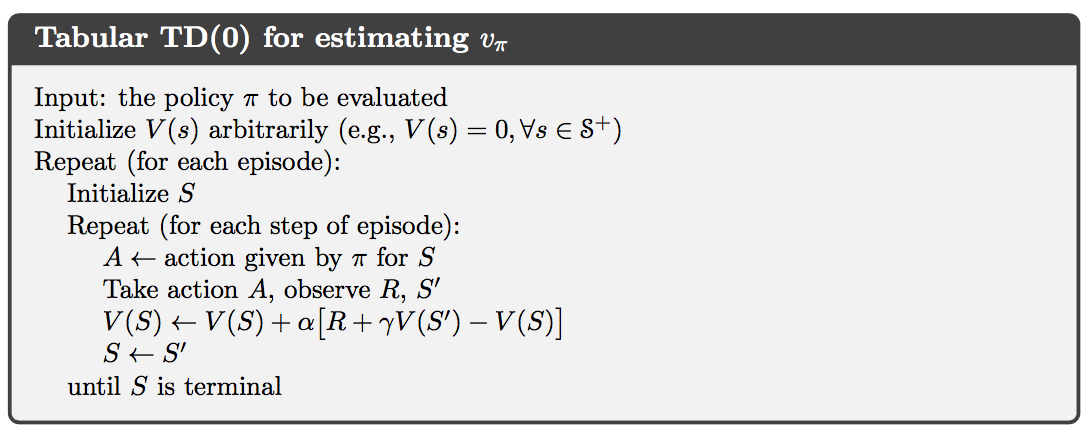Note that the quantity in brackets in the TD(0) update is a sort of error, measuring the difference between the estimated value of $S_t$ and the better estimate $R_t+\gamma V(S_{t+1})$. This quantity, called the TD error, arises in various forms throughout reinforcement learning:

Also note that the Monte Carlo error can be written as a sum of TD errors:

This fact and its generalizations play important roles in the theory of TD learning.

### Optimality of TD(0)

Suppose there is available only a finite amount of experience, say 10 episodes or 100 time steps. In this case, a common approach with incremental learning methods is to present the experience repeatedly until the method converges upon an answer. Updates are made only after processing each complete batch of training data. We call this batch updating.

Batch Monte Carlo methods always find the estimates that minimize mean-squared error on the training set, whereas batch TD(0) always finds the estimates that would be exactly correct for the maximum-likelihood model of the Markov process. In this case, the maximum-likelihood estimate is the model of the Markov process formed in the obvious way from the observed episodes: the estimated transition probability from $i$ to $j$ is the fraction of observed transitions from $i$ that went to $j$, and the associated expected reward is the average of the rewards observed on those transitions. Given this model, we can compute the estimate of the value function that would be exactly correct if the model were exactly correct. This is called the certainty-equivalence estimate because it is equivalent to assuming that the estimate of the underlying process was known with certainty rather than being approximated. In general, batch TD(0) converges to the certainty-equivalence estimate.

### Sarsa: On-Policy TD Control

As usual, we follow the pattern of generalized policy iteration (GPI), only this time using TD methods for the evaluation or prediction part.

In the previous section we considered transitions from state to state and learned the values of states. Now we consider transitions from state–action pair to state–action pair, and learn the values of state–action pairs. The theorems assuring the convergence of state values under TD(0) also apply to the corresponding algorithm for action values: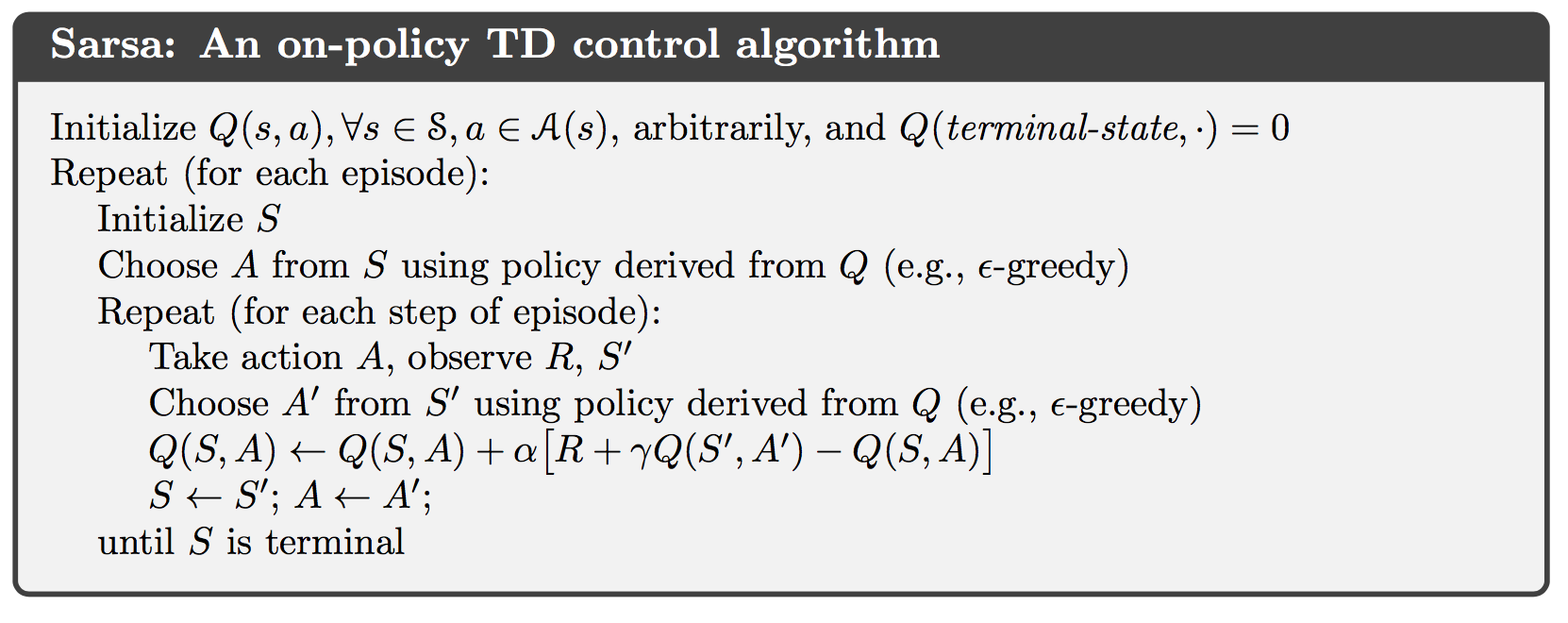### Q-learning: Off-Policy TD Control

One of the early breakthroughs in reinforcement learning was the development of an off-policy TD control algorithm known as Q-learning, defined by:

In this case, the learned action-value function, $Q$, directly approximates $q^*$, the optimal action-value function, independent of the policy being followed.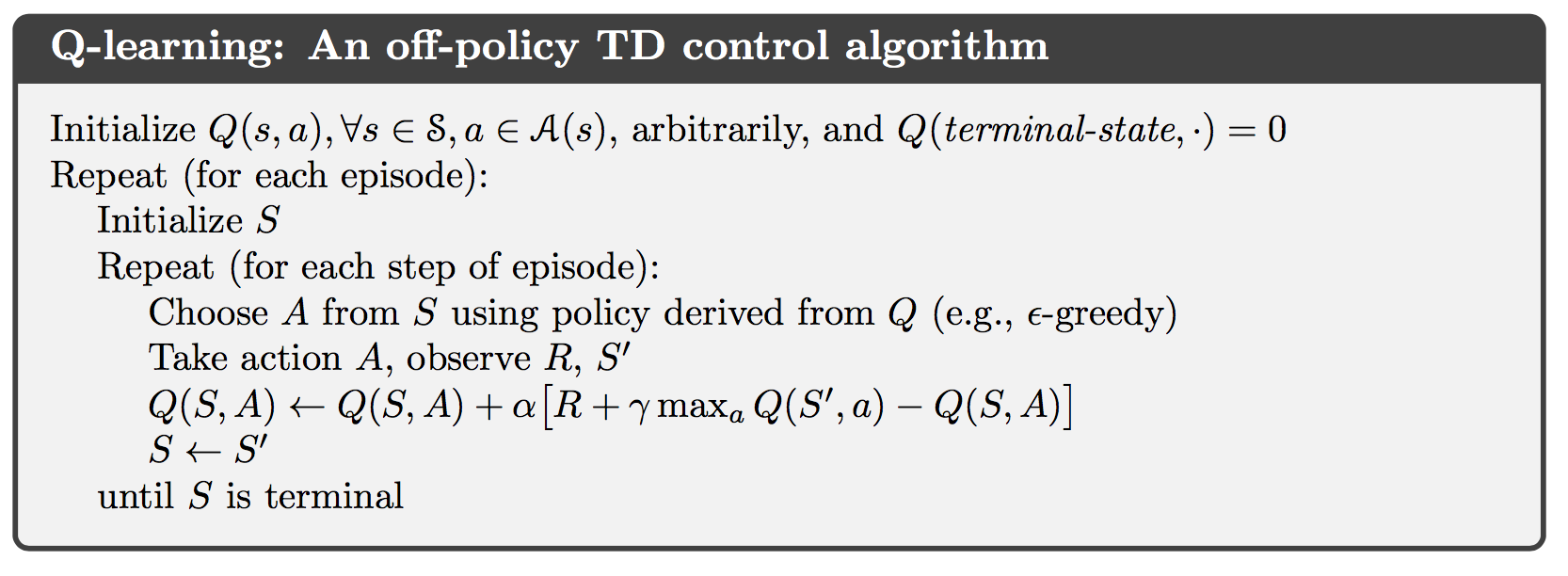### Expected Sarsa

Consider the algorithm with the update rule:

but that otherwise follows the schema of Q-learning. Given the next state $S_{t+1}$, this algorithm moves deterministically in the same direction as Sarsa moves in expectation, and accordingly it is called expected Sarsa.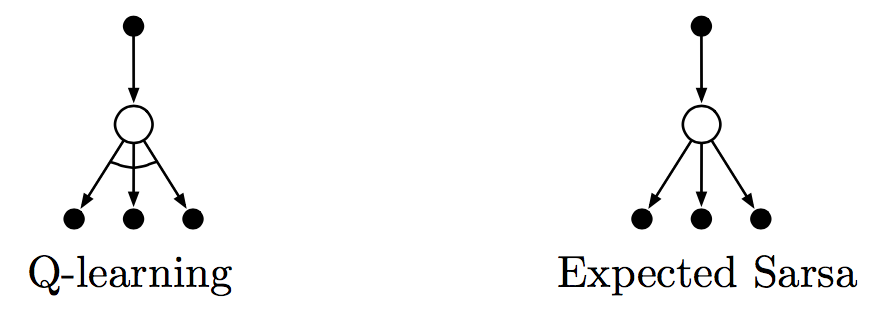### Maximization Bias and Double Learning

All the control algorithms that we have discussed so far involve maximization in the construction of their target policies. In these algorithms, a maximum over estimated values is used implicitly as an estimate of the maximum value, which can lead to a significant positive bias. We call this maximization bias.

One way to view the problem is that it is due to using the same samples (plays) both to determine the maximizing action and to estimate its value. Suppose we divided the plays in two sets and used them to learn two independent estimates, call them $Q_1(a)$ and $Q_2(a)$, each an estimate of the true value $q(a)$, for all $a\in\mathcal{A}$. We could then use one estimate, say $Q_1$, to determine the maximizing action $A^*=\mathrm{argmax}_aQ_1(a)$, and the other, $Q_2$, to provide the estimate of its value, $Q_2(A^*)=Q_2(\mathrm{argmax}_aQ_1(a))$. This estimate will then be unbiased in the sense that $\mathbb{E}[Q_2(A^*)]=q(A^*)$. We can also repeat the process with the role of the two estimates reversed to yield a second unbiased estimate $Q_1(A^*)=Q_1(\mathrm{argmax}_aQ_2(a))$. This is the idea of doubled learning.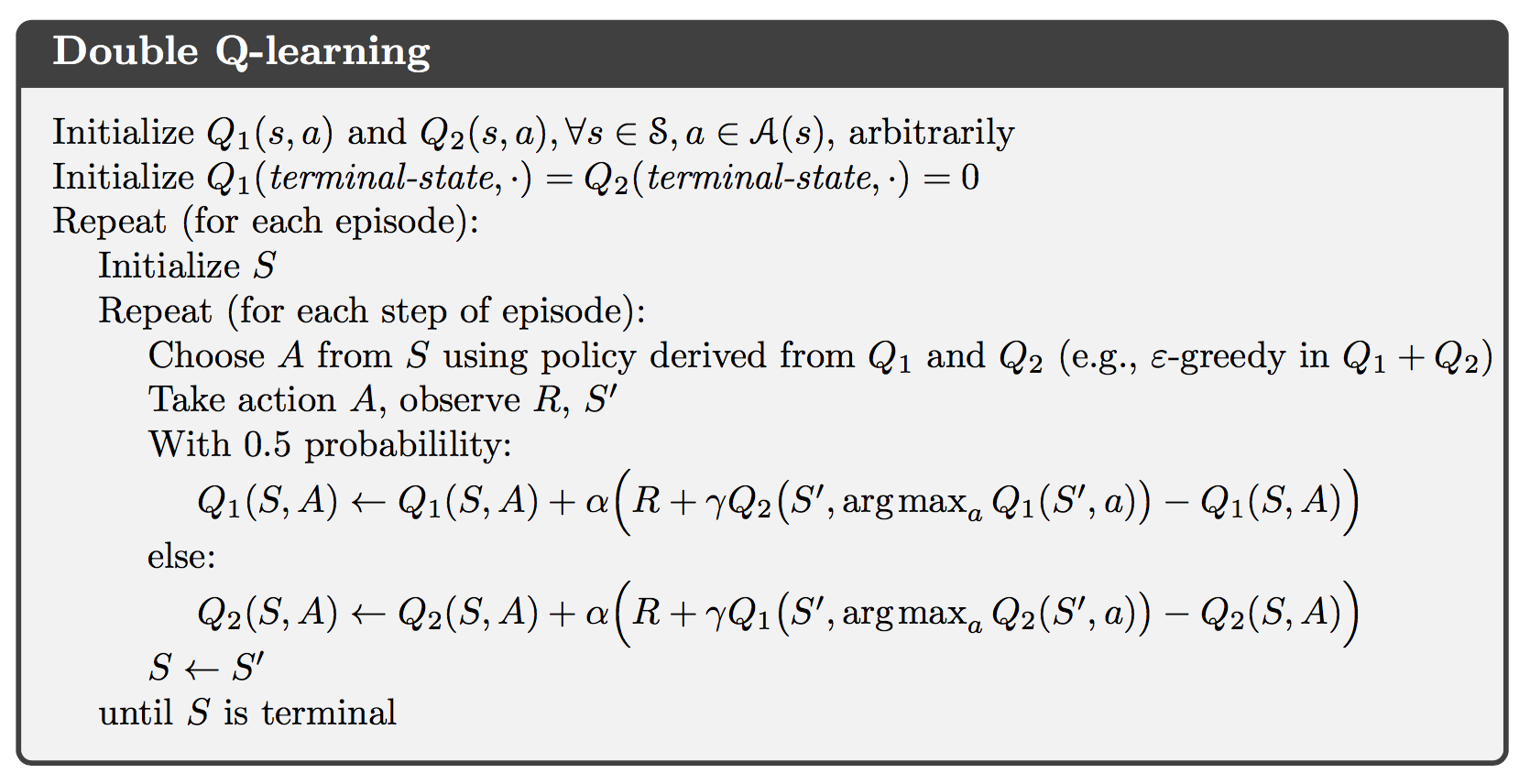]]>
<![CDATA[Notes on Reinforcement Learning (3): Monte Carlo Methods]]> 2016-10-14T18:07:35-04:00 http://billy-inn.github.io/blog/2016/10/14/notes-on-reinforcement-learning-3-monte-carlo-methods Monte Carlo methods are ways of solving the reinforcement learning problem based on averaging sample returns. To ensure that well-defined returns are available, here we define Monte Carlo methods only for episodic tasks.

### Monte Carlo Prediction

An obvious way to estimate the state-value function which is the expected return starting from that state from experience, is to average the returns observed after visits to that state. This idea underlies all Monte Carlo methods.

In particular, suppose we wish to estimate $v_\pi(s)$, the value of a state $s$ under policy $\pi$, given a set of episodes obtained by following $\pi$ and passing through $s$. Each occurrence of state $s$ in an episode is called a visit to $s$. The first-visit MC method estimates $v_\pi(s)$ as the average of the returns following from first visits to $s$, whereas every-visit MC method averages the returns following all visits to $s$.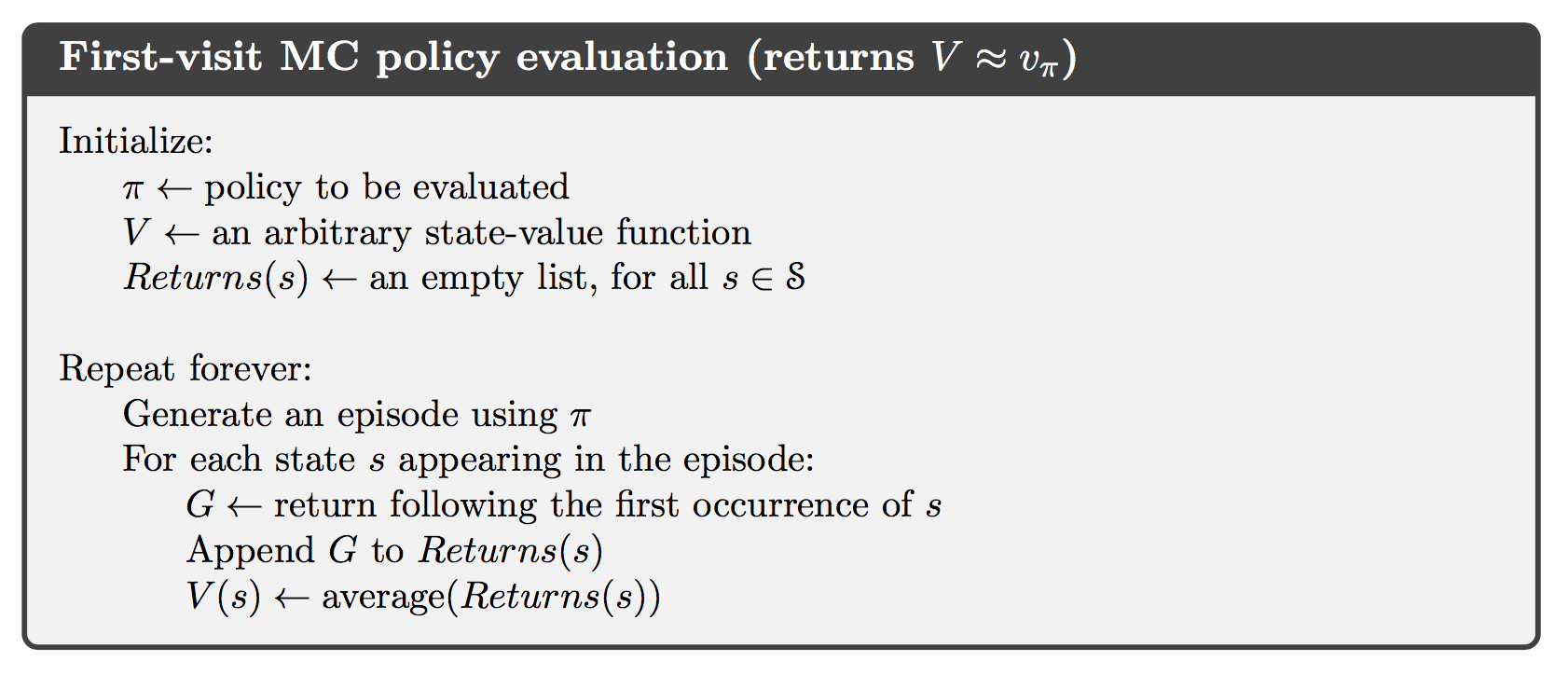An important fact about Monte Carlo method methods is that the estimates for each state are independent. The estimate for one state does not build upon the estimate of any other state, as is the case in DP.

### Monte Carlo Estimation of Action Values

If a model is not available, then it is particularly useful to estimate action values rather than state values. The Monte Carlo methods for this this are essentially the same as just presented for state values, except now we talk about visits to a state–action pair rather than to a state. A state–action pair $s, a$ is said to be visited in an episode if ever the state $s$ is visited and action $a$ is taken in it.

The only complication is that many state–action pairs may never be visited. This is the general problem of maintaining exploration.

### Monte Carlo Control

The overall idea of how Monte Carlo estimation can be used in control is to according to the idea of generalized policy iteration (GPI). In GPI one maintains both an approximate policy and an approximate value function. The value function is repeatedly altered to more closely approximate the value function for the current policy, and the policy is repeatedly improved with respect to the current value function.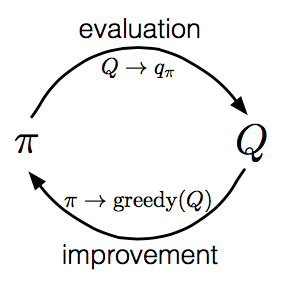We made two unlikely assumptions in order to easily obtain guarantee of convergence for the Monte Carlo method. One was that the episodes have exploring starts, and the other was that policy evaluation could be done with an infinite number of episodes.

The assumption that policy evaluation operates on an infinite number of episodes are relatively easy to remove. One of the approaches it to forgo trying to complete policy evaluation before returning to policy improvement. For Monte Carlo policy evaluation it is natural to alternate between evaluation and improvement on an episode-by-episode basis.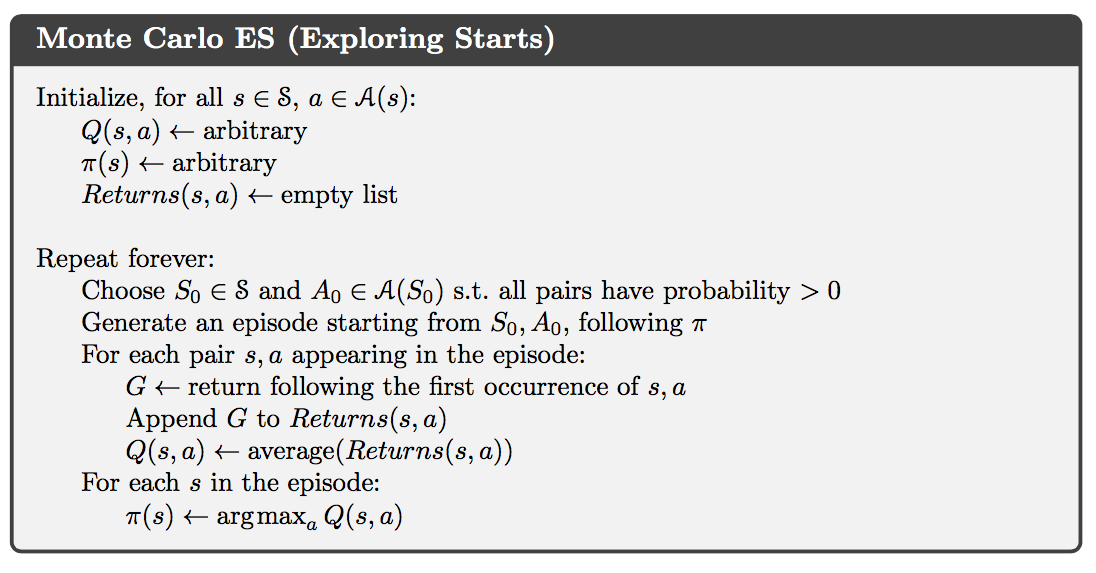### Monte Carlo Control without Exploring Starts

The only general way to ensure that all actions are selected infinitely often is for the agent to continue to select them. There are two approaches to ensuring this, resulting in what we call on-policy methods and off-policy methods. On-policy methods attempt to evaluate or improve the policy that is used to make decisions, whereas on-policy methods evaluate or improve a policy different from that used to generate the data.

In on-policy control methods the policy is generally soft, meaning that $\pi(a \vert s)>0$ for all $s\in\mathcal{S}$ and all $a\in\mathcal{A}(s)$, but gradually shifted closer and closer to a deterministic optimal policy.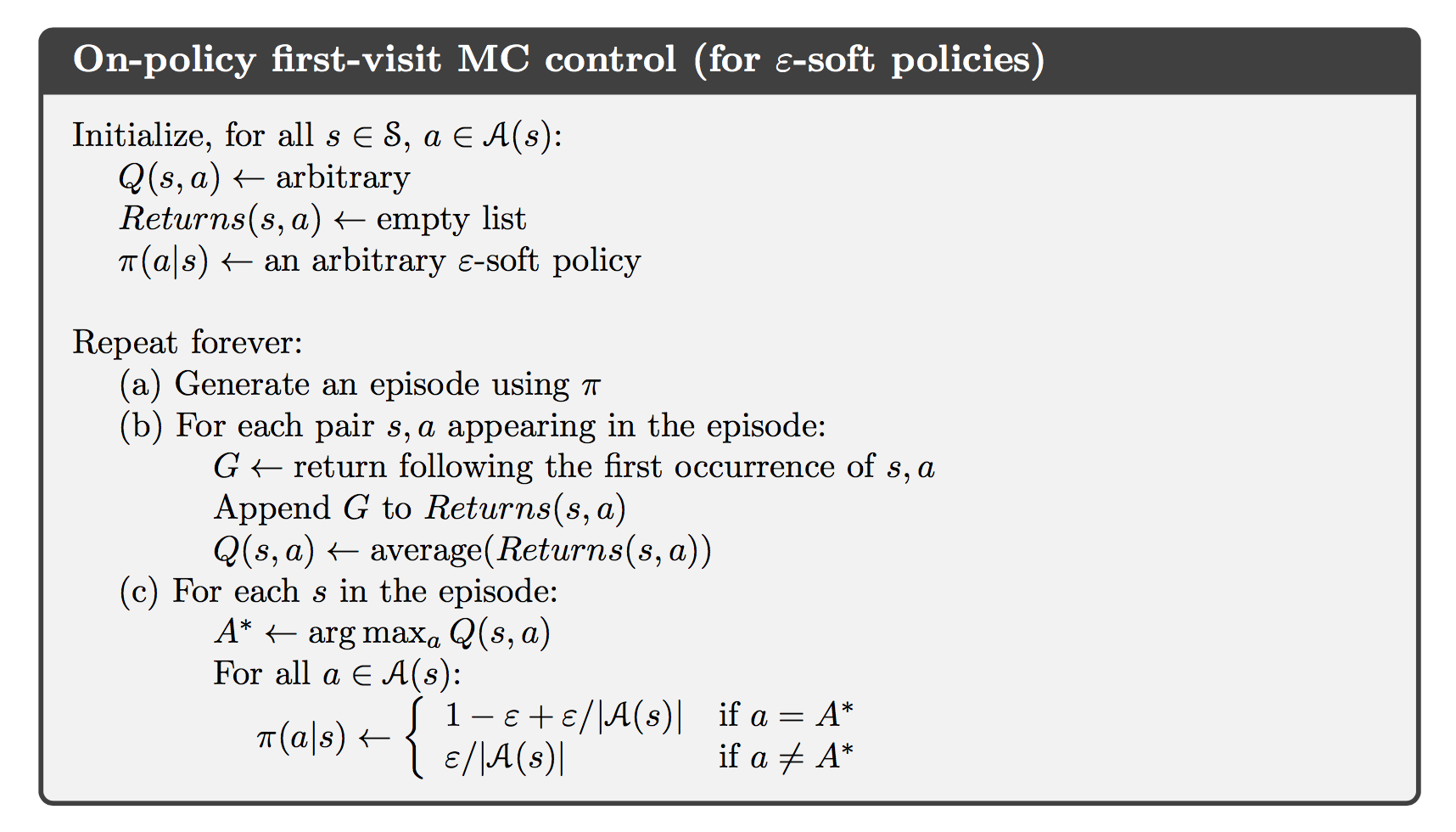### Off-policy Prediction via Importance Sampling

All learning control methods face a dilemma: They seek to learn action values conditional on subsequent optimal behavior, but they need to behave non-optimally in order to explore all actions (to find the optimal actions). How can they learn about the optimal policy while behaving according to an exploratory policy? The on-policy approach in the preceding section is actually a compromise. It learns action values not for the optimal policy, but for a near-optimal policy that still explores. A more straightforward approach is to use two policies, one that is learned about and that becomes the optimal policy, and one that is more exploratory and is used to gen- erate behavior. The policy being learned about is called the target policy, and the policy used to generate behavior is called the behavior policy. In this case we say that learning is from data “off” the target policy, and the overall process is termed off-policy learning.

Suppose we wish to estimate $v_\pi$ or $q_\pi$, but we have all we have are episodes following another policy $\mu$, where $\mu \ne \pi$. In this case, $\pi$ is the target policy, $\mu$ is the behavior policy, and both policies are considered fixed and given. We require that $\pi(a \vert s)>0$ implies $\mu(a \vert s)>0$. This is called the assumption of coverage.

Almost all off-policy methods utilize importance sampling, a general technique for estimating expected values under one distribution given samples from another. Given a starting state $S_t$, the probability of the subsequent state-action trajectory, $A_t,S_{t+1},A_{t+1},\dots,S_T$, occurring under any policy $\pi$ is

Thus, the relatvie probability of the trajectory under the target and behavior policies (the importance-sampling ratio) is

We can define the set of all time steps in which state is visited, denoted $\mathcal{J}(s)$. This is for an every-visit method; for a first-visit method, $\mathcal{J}(s)$ would only include time steps that were first visits to $s$ within their episodes. Also, let $T(t)$ denote the first time of termination following time $t$, and $G_t$ denote the return after $t$ up through $T(t)$. Then $\{G_t\}_{t\in\mathcal{J}(s)}$ are the returns that pertain to state $s$, and $\{\rho_t^{T(t)}\}_{t\in\mathcal{J}(s)}$ are the corresponding importance-sampling ratios. To estimate $v_{\pi}(s)$, we simply scale the returns by the ratios and average the results:

When importance sampling is done as a simple average in this way it is called ordinary importance sampling.

An import alternative iis weighted importance sampling, which uses a weighted average, defined as

or zero if the denominator is zero.

The difference between the two kinds of importance sampling is expressed in their biases and variances. The ordinary importance-sampling estimator is unbiased whereas the weighted importance-sampling estimator is biased. On the other hand, the variance of the ordinary importance-sampling estimator is in general unbounded because the variance of the ratios can be unbounded, whereas in the weighted estimator the largest weight on any single return is one. In fact, assuming bounded returns, the variance of the weighted importance-sampling estimator converges to zero even if the variance of the ratios themselves is infinite. In practice, the weighted estimator usually has dramatically lower variance and is strongly preferred.

### Incremental Implementation

Suppose we have a sequence of returns $G_1, G_2, \dots, G_{n-1}$, all starting in the same state and each with a corresponding random weight $W_i$ (e.g., $W_i=\rho_t^{T(t)}$).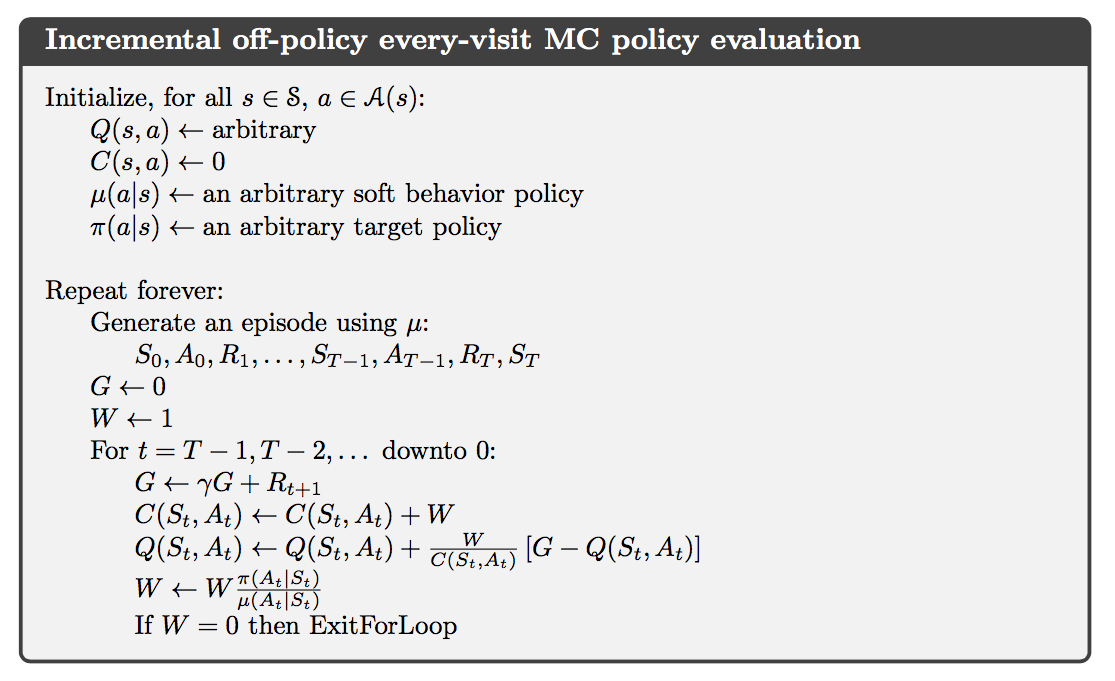### Off-Policy Monte Carlo Control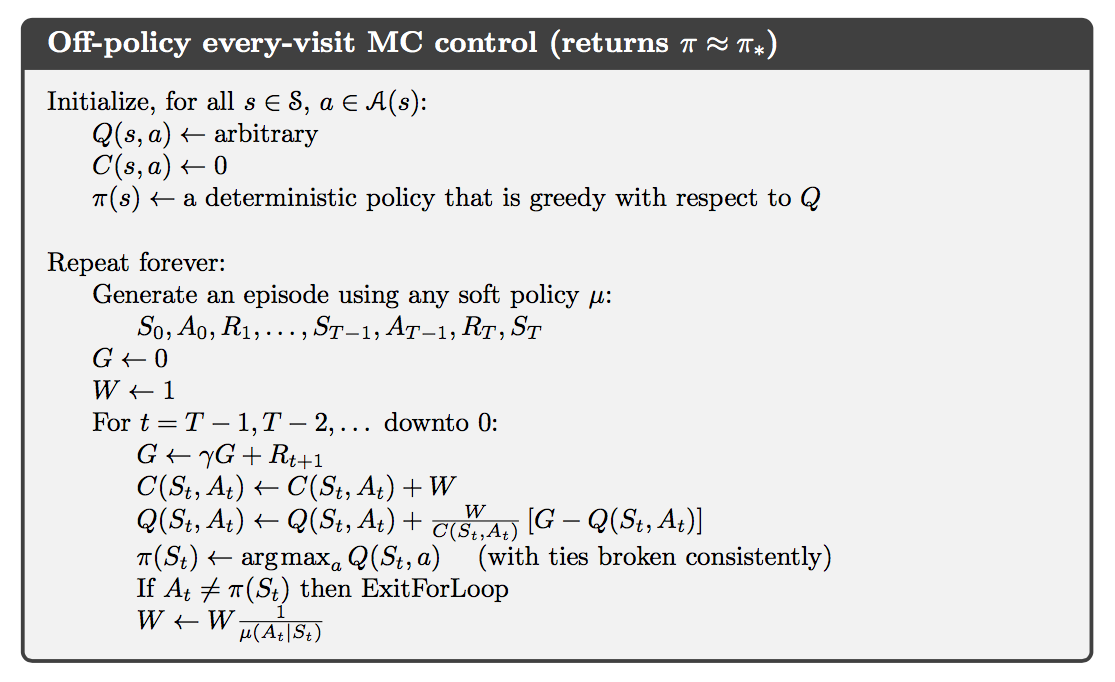]]>
<![CDATA[Notes on Reinforcement Learning (2): Dynamic Programming]]> 2016-10-06T19:37:02-04:00 http://billy-inn.github.io/blog/2016/10/06/notes-on-reinforcement-learning-2-dynamic-programming Policy Evaluation

Consider a sequence of approximate value functions $v_0, v_1, v_2, \dots,$ each mapping $\mathcal{S}^+$ to $\mathbb{R}$. The initial approximation, $v_0$ is chosen arbitrarily, and each successive approximation is obtained by using the Bellman equation for $v_\pi$ as an update rule:

for all $s\in\mathcal{S}$.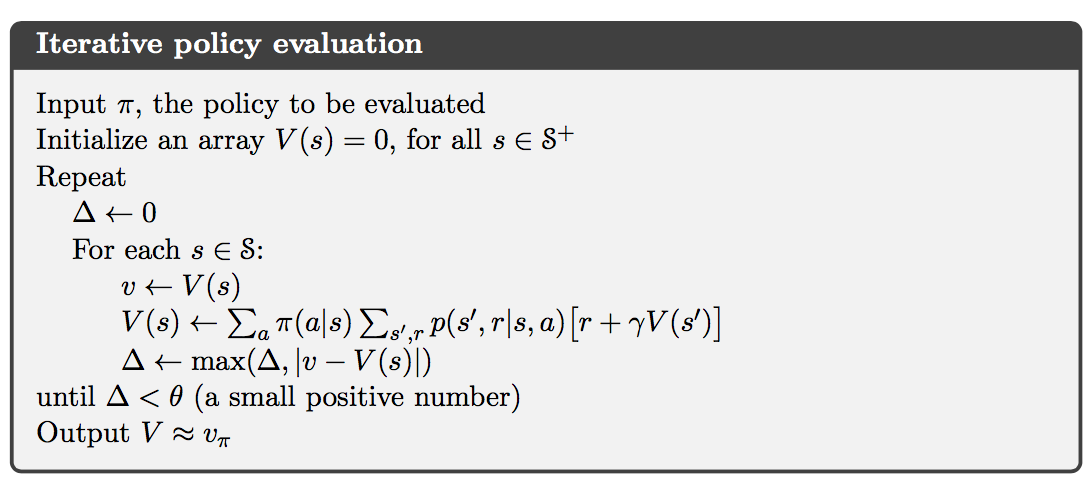### Policy Improvement

Let $\pi$ and $\pi’$ be any pair of deterministic policies such that, for all $s\in\mathcal{S}$,

Then the policy $\pi’$ must be as good as, or better than, $\pi$. That is, it must obtain greater or equal expected return from all states $s\in\mathcal{S}$:

This result is called policy improvement theorem.

Consider the new greedy policy, $\pi’$, given by

The greedy policy takes the action that looks best in the short term according to $v_\pi$. By construction, the greedy policy meets the conditions of the policy improvement theorem. The process of making a new policy that improves on an original policy, by making it greedy with respect to the value function of the original policy, is called policy improvement.

In addition, policy improvement must give us a strictly better policy excepy when the original policy is already optimal.

### Policy Iteration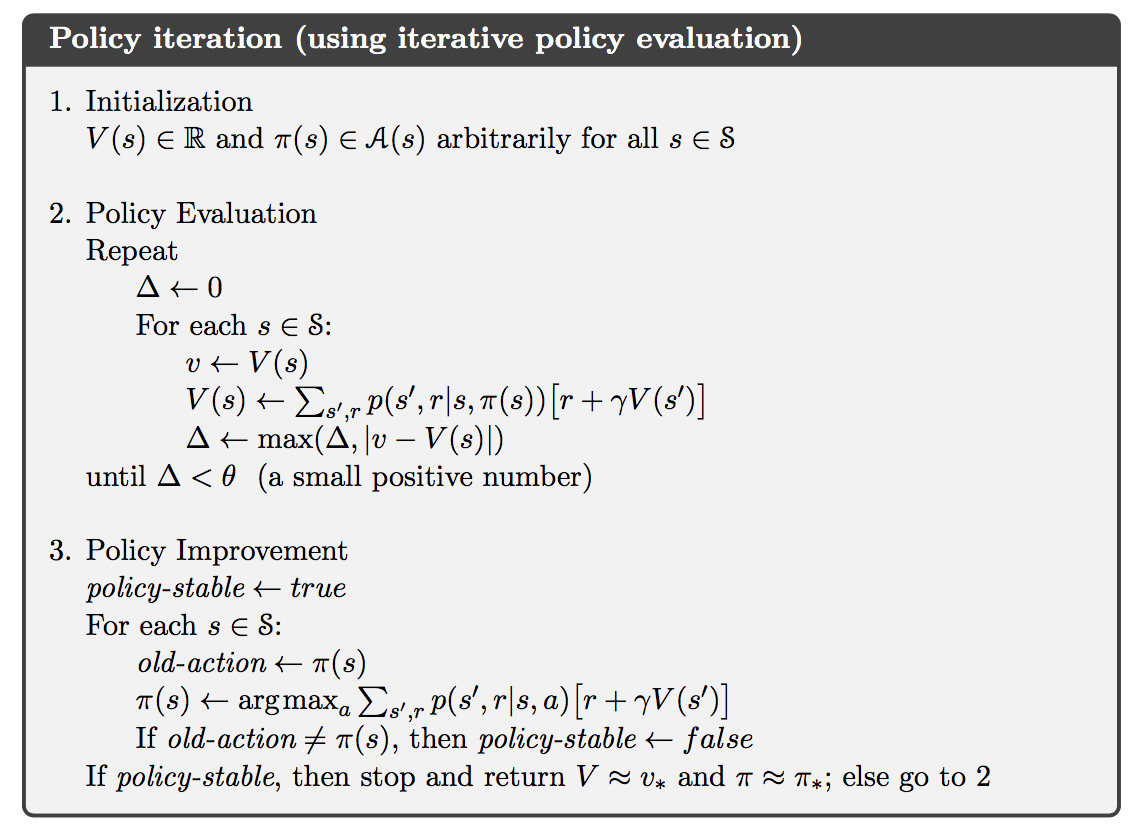### Value Iteration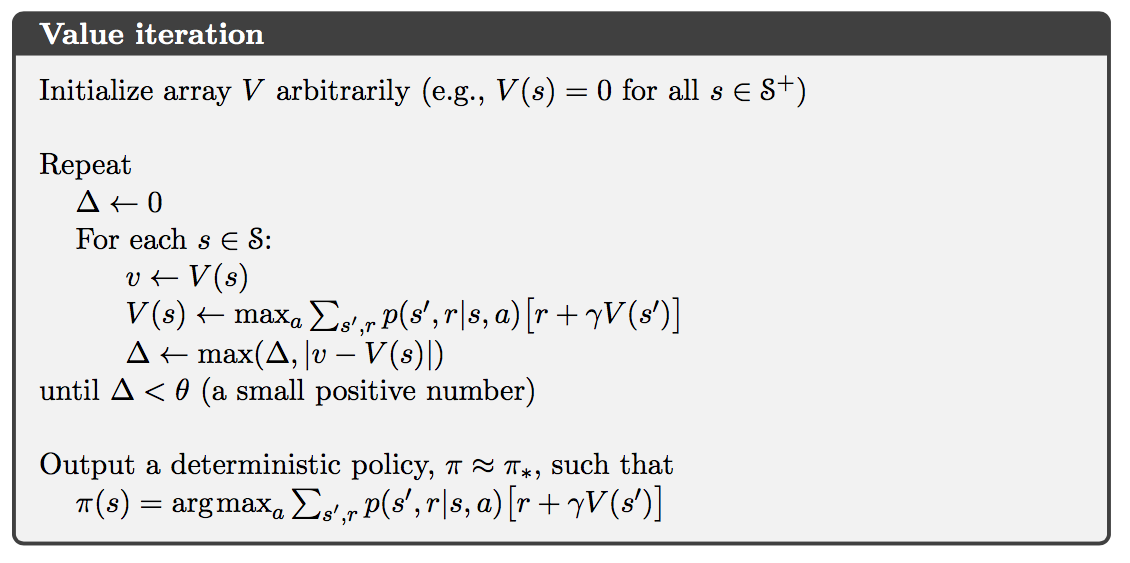### Generalized Policy Iteration

We use the term generalized policy iteration (GPI) to refer to the general idea of letting policy evaluation and policy improvement processes interact, independent of the grandularity and other details of the two processes.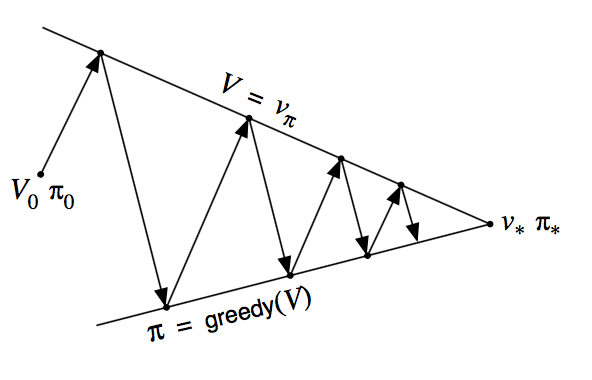]]>
<![CDATA[Notes on Reinforcement Learning (1): Finite Markov Decision Processes]]> 2016-10-05T16:55:31-04:00 http://billy-inn.github.io/blog/2016/10/05/notes-on-reinforcement-learning-1-finite-markov-decision-processes The Agent-Environment Interface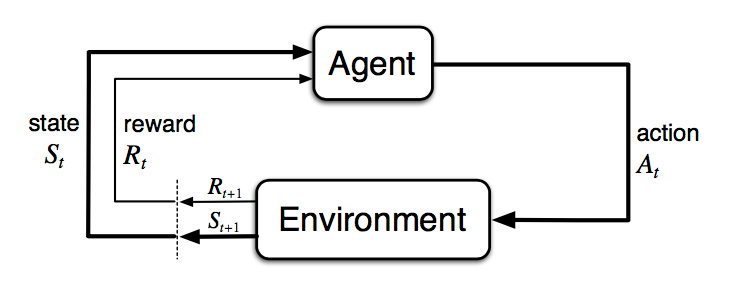• The agent and environment interact at each of a sequence of discrete time steps, $t=0,1,2,3,\dots$
• At each time step $t$, the agent receives some representation of the environment’s state, $S_t\in\mathcal{S}$, where $\mathcal{S}$ is the set of possible states.
• On that basis, the agent selects an action, $A_t \in \mathcal{A}(S_t)$, where $\mathcal{A}(S_t)$ is the set of actions available in state $S_t$.
• One time step later, in part as a consequence of its action, the agent receives a numerical reward, $R_{t+1} \in \mathcal{R} \subset \mathbb{R}$, and finds itself in a new state, $S_{t+1}$.

At each time step, the agent implements a mapping from states to probabilities of selecting each possible action. This mapping is called the agent’s policy and is denoted $\pi_t$, where $\pi_t(a \vert s)$ is the probability that $A_t=a$ if $S_t=s$. Reinforcement learning methods specify how the agent changes its policy as a result of its experience. The agent’s goal, roughly speaking, is to maximize the total amount of reward it receives over the long run.

The general rule we follow is that anything that cannot be changed arbitrarily by the agent is considered to be outside of it and thus part of its environment.

### Rewards and Returns

At each time step, the reward is a simple number, $R_t \in \mathbb{R}$. Informally, the agent’s goal is to maximize the total amount of reward it receives. If the sequence of rewards received after time step $t$ is denoted $R_{t+1}, R_{t+2}, R_{t+3}, \dots$, we seek to maximize the expected return, where the return $G_t$ is defined as some specific function of the reward sequence.

Here we define the return as:

where $T$ can be $\infty$ and $0<\gamma\le1$ is the discounting rate.

### Markov Decision Processes

A reinforment learning task that satisfies the Markov preperty is called a Markov decision process, or MDP. If the state and action spaces are finite, then it is called a finite Markov decision process (finite MDP).

Given any state and action $s$ and $a$, the probability of each possible pair of next state and reward, $s’$, $r$, is denoted

These quantities completely specify the dynamics of a finite MDP.

### Value Functions

The value of a state $s$ under a policy $\pi$, denoted $v_\pi(s)$, is the expected return when starting in $s$ and following $\pi$ thereafter. For MDPs, we can define $v_\pi(s)$ formally as

where $\mathbb{E}[\centerdot]$ denotes the expected value of a random variable given that the agent follows policy $\pi$, and $t$ is any time step. We call the function $v_\pi$ the state-value function for policy $\pi$.

Similarly, we define the value of taking action $a$ in state $s$ under a policy $\pi$, denoted $q_\pi(s,a)$, as the expected return starting from $s$, taking the action $a$, and thereafter following policy $\pi$:

We call $q_\pi$ the action-value function for policy $\pi$.

A fundamental property of value functions used throughout reinforcement learning and dynamic programming is that they satisfy particular recursive relationships. For any policy $\pi$ and any state $s$, the following consistency condition holds between the value of $s$ and the value of its possible successor states:

This equation is the Bellman equation for $v_\pi$. Likewise, the Bellman equation for action values $q_\pi$ is as follows: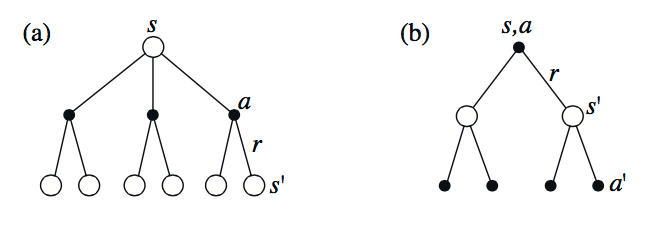According to the Bellman equations, we can derive the relatioship between $v_\pi$ and $q_\pi$:

### Optimal Value Functions

A policy $\pi$ is defined to be better than or equal to a policy $\pi’$ if its expected return is greater than or equal to that of $\pi’$ for all states. In other words, $\pi\ge\pi’$ if and only if $v_\pi(s)\ge v_{\pi’}(s)$ for all $s\in\mathcal{S}$. There is always at least one policy that is better than or equal to all other policies. This is an optimal policy $\pi_*$.

The optimal state-value function, denoted $v_*$, are defined as

for all $s\in\mathcal{S}$.

The optimal action-value function, denoted $q_*$, are defined as

for all $s\in\mathcal{S}$ and $a\in\mathcal{A}(s)$.

The Bellman optimalality equation for $v_$ and $q_$ is

]]>